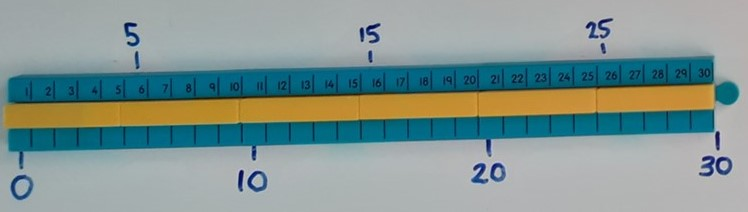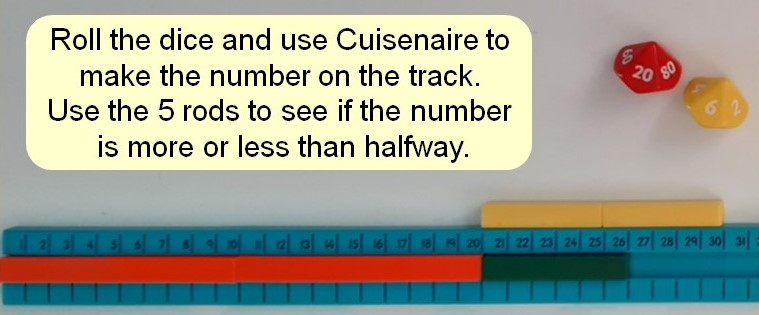## Rounding to the nearest 10

The first step in rounding numbers is to understand the position of numbers on a numberline. Using a number track and Cuisenaire rods allows a pupil to see which multiples of 10 a number lies between and to explore the numbers that are halfway between the multiples, recognising that the halfway numbers always end in 5 ones.Pupils can then practise placing numbers between the correct multiples of 10 on an empty numberline, estimating the position by considering which multiple of 10 the number is closest too. We then say that the number rounds to that multiple. When a number is exactly halfway between the multiples, we always round this up to the next multiple of 10.

74 has less than 5 ones, meaning it is closer to 70 than 80, so it rounds down to 7077 has more than 5 ones, meaning it is closer to 80 than 70, so it rounds up to 80.75 has 5 ones, meaning it is exactly halfway between multiples, so it also rounds up to the next multiple of 10.### Summary

When rounding to the nearest 10 we check the ones digit:

If the ones digit is less than 5, we round down to the previous multiple of 10. The tens digit stays the same and we replace any ones with a zero.

If the ones digit is 5 or more, we round up to the next multiple of 10. We add 1 to the tens digit and we replace any ones with a zero.

After rounding to 10, the number will always end in a zero.

Play Rounding race to practice rounding to the nearest 10.